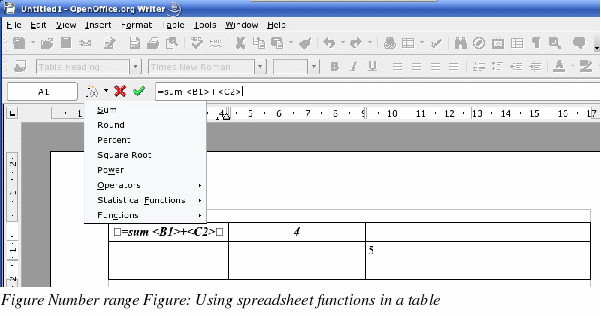On-line GuidesAll GuideseBook StoreiOS / AndroidLinux for BeginnersOffice ProductivityLinux InstallationLinux SecurityLinux UtilitiesLinux VirtualizationLinux KernelSystem/Network AdminProgrammingScripting LanguagesDevelopment ToolsWeb DevelopmentGUI Toolkits/DesktopDatabasesMail SystemsopenSolarisEclipse DocumentationTechotopia.comVirtuatopia.comHow To GuidesVirtualizationGeneral System AdminLinux SecurityLinux FilesystemsWeb ServersGraphics & DesktopPC HardwareWindowsProblem SolutionsPrivacy PolicyOpenOffice Writer User Guide
Previous Page Home Next Page

# Using spreadsheet functions in a table

In a table in a Writer document, you can use some of the mathematical functions that are normally implemented by OpenOffice.org Calc. For many simpler functions, Writer tables can be used as a basic spreadsheet.

Just as in a spreadsheet, each table cell is identified by a letter (for the column) and a number (for the row). For example, cell C4 is the cell in the third column from the left and fourth row from the top. When the cursor is in a cell, this cell reference is displayed on the status bar (in its default position at the bottom right corner of the screen).

 TIP Basic spreadsheet functions in tables are much the same as in OpenOffice.org Calc. The main difference is that cell references are formatted differently. Cell A2 (first column, second row) is referred to in Calc as A2 (or \$A\$2 for an absolute reference). In Writer tables, it is referred to as .

For example, suppose you had two numbers in cells <B1> and <C2> and wanted to display the sum of the two in cell <A1>, as shown in Figure 256.

Do the following:

1. Click in cell <A1> and press the = key. The Formula bar appears automatically, near the top of the screen. In the leftmost side of the bar, you can see the coordinates of the selected cell.

2. Click in cell <B1>. The identifiers of this cell are automatically displayed in the Formula bar and inserted into cell <A1>.

3. Press the + key.

4. Click on cell <C2>. You can see the final formula â€œ= <B1>+<C2>â€ displayed both in the selected cell and in the Object bar.

5. Press the Enter key or click the green tick on the Formula Bar.TIP To display the list of the mathematical functions that you can use in a table: Display the Formula toolbar by pressing F2 or select a blank cell and press the = key. Click and hold the Formula f(x) icon.

In our example, this gives the result 9 in the top left cell. For contiguous cells, you can simply select the cells in the row, column, or the rectangle of rows and columns. Thus, for example, to add a column of numbers, do this:

1. Type an equals sign â€˜=â€™ in an empty cell.

2. Select the cells to be added together, in this case the cells from A2 to A5. The formula should be something like â€œ=<A2:A5>â€.

3. Press the Enter key or click the green tick on the Formula Bar.

4. The answer appears in the cell you have selected.

When using a function, you can enter the cells manually or by selecting them. Thus, to add up the four numbers that we added above (A2, A3, A4, A5), do this:

1. Type an equals sign â€˜=â€™ in an empty cell.

2. Type â€˜sumâ€™ or select it from the function list f(x).

3. Select the cells to be added together. The formula should be something like â€œ=sum<A2:A5>â€.

4. Press the Enter key or click the green tick on the Formula Bar.

5. The answer appears in the cell you have selected.OpenOffice Writer User Guide
Previous Page Home Next PagePublished under the terms of the Open Publication License Design by Interspire